NCERT Exemplar Class 10 Science Chapter 12: Electricity. NCERT Exemplar Solutions for Class 10 Science Chapter 12 Electricity prepare students for their Class 10 exams thoroughly.

Science problems and solutions for the Class 10 pdf are provided here which are similar to the questions being asked in the previous year’s board.

## NCERT Exemplar Class 10 Science Chapter 12: Electricity

Class 10 : Science Chapter 12 solutions. Complete Class 10 Science Chapter 12 Notes.

Multiple Choice Questions

1. A cell, a resistor, a key and ammeter are arranged as shown in the circuit diagrams of Figure 12.1. The current recorded in the ammeter will be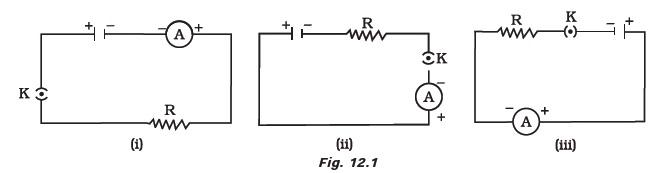• (a) maximum in (i)
• (b) maximum in (ii)
• (c) maximum in (iii)
• (d) the same in all the cases
2. In the following circuits (Figure 12.2), heat produced in the resistor or combination of resistors connected to a 12 V battery will be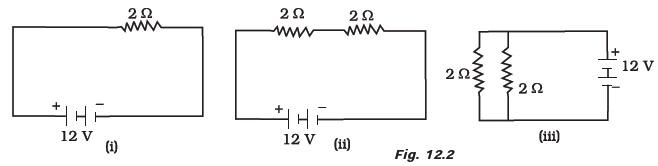• (a) same in all the cases
• (b) minimum in case (i)
• (c) maximum in case (ii)
• (d) maximum in case (iii)
3. Electrical resistivity of a given metallic wire depends upon
• (a) its length
• (b) its thickness
• (c) its shape
• (d) nature of the material
4. A current of 1 A is drawn by a filament of an electric bulb. Number of electrons passing through a cross section of the filament in 16 seconds would be roughly
• (a) 1020
• (b) 1016
• (c) 1018
• (d) 1023
5. Identify the circuit (Figure 12.3) in which the electrical components have been properly connected.• (a) (i)
• (b) (ii)
• (c) (iii)
• (d) (iv)
6. What is the maximum resistance which can be made using five resistors each of 1/5 Ω?
• (a) 1/5 Ω
• (b) 10 Ω
• (c) 5 Ω
• (d) 1 Ω
7. What is the minimum resistance which can be made using five resistors each of 1/5 Ω?
• (a) 1/5 Ω
• (b) 1/25 Ω
• (c) 1/10 Ω
• (d) 25 Ω
8. The proper representation of series combination of cells (Figure 12.4) obtaining maximum potential is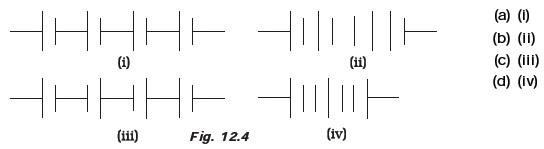• (a) (i)
• (b) (ii)
• (c) (iii)
• (d) (iv)
9. Which of the following represents voltage?10. A cylindrical conductor of length l and uniform area of cross section A has resistance R. Another conductor of length 2l and resistance R of the same material has area of cross section
• (a) A / 2
• (b) 3A / 2
• (c) 2A
• (d) 3A
11. A student carries out an experiment and plots the V-I graph of three samples of nichrome wire with resistances R1, R2 and R3 respectively (Figure.12.5). Which of the following is true?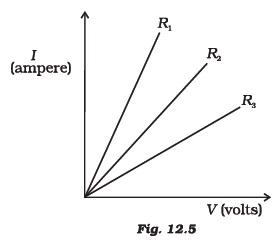• (a) R1 = R2 = R3
• (b) R1 > R2 > R3
• (c) R3 > R2 > R1
• (d) R2 > R3 > R1
12. If the current I through a resistor is increased by 100% (assume that temperature remains unchanged), the increase in power dissipated will be
• (a) 100 %
• (b) 200 %
• (c) 300 %
• (d) 400 %
13. The resistivity does not change if
• (a) the material is changed
• (b) the temperature is changed
• (c) the shape of the resistor is changed
• (d) both material and temperature are changed
14. In an electrical circuit three incandescent bulbs A, B and C of rating 40 W, 60 W and 100 W respectively are connected in parallel to an electric source. Which of the following is likely to happen regarding their brightness?
• (a) Brightness of all the bulbs will be the same
• (b) Brightness of bulb A will be the maximum
• (c) Brightness of bulb B will be more than that of A
• (d) Brightness of bulb C will be less than that of B
15. In an electrical circuit two resistors of 2 Ω and 4 Ω respectively are connected in series to a 6 V battery. The heat dissipated by the 4 Ω resistor in 5 s will be
• (a) 5 J
• (b) 10 J
• (c) 20 J
• (d) 30 J
16. An electric kettle consumes 1 kW of electric power when operated at 220 V. A fuse wire of what rating must be used for it?
• (a) 1 A
• (b) 2 A
• (c) 4 A
• (d) 5 A
17. Two resistors of resistance 2 Ω and 4 Ω when connected to a battery will have
• (a) same current flowing through them when connected in parallel
• (b) same current flowing through them when connected in series
• (c) same potential difference across them when connected in series
• (d) different potential difference across them when connected in parallel
18. Unit of electric power may also be expressed as
• (a) volt ampere
• (b) kilowatt hour
• (c) watt second
• (d) joule second

1. A child has drawn the electric circuit to study Ohm’s law as shown in Figure 12.6. His teacher told that the circuit diagram needs correction. Study the circuit diagram and redraw it after making all corrections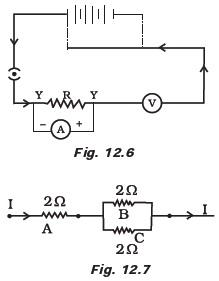2. Three 2 Ω resistors, A, B and C, are connected as shown in Figure 12.7. Each of them dissipates energy and can withstand a maximum power of 18W without melting. Find the maximum current that can flow through the three resistors?
3. Should the resistance of an ammeter be low or high? Give reason.
4. Draw a circuit diagram of an electric circuit containing a cell, a key, an ammeter, a resistor of 2 Ω in series with a combination of two resistors (4 Ω each) in parallel and a voltmeter across the parallel combination. Will the potential difference across the 2 Ω resistor be the same as that across the parallel combination of 4Ω resistors? Give reason.
5. How does use of a fuse wire protect electrical appliances?
6. What is electrical resistivity? In a series electrical circuit comprising a resistor made up of a metallic wire, the ammeter reads 5 A. The reading of the ammeter decreases to half when the length of the wire is doubled. Why?
7. What is the commercial unit of electrical energy? Represent it in terms of joules.
8. A current of 1 ampere flows in a series circuit containing an electric lamp and a conductor of 5 Ω when connected to a 10 V battery. Calculate the resistance of the electric lamp.
Now if a resistance of 10 Ω is connected in parallel with this series combination, what change (if any) in current flowing through 5 Ω conductor and potential difference across the lamp will take place? Give reason.
9. Why is parallel arrangement used in domestic wiring?
10. B1, B2 and B3 are three identical bulbs connected as shown in Figure 12.8. When all the three bulbs glow, a current of 3A is recorded by the ammeter A.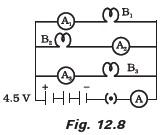• (i) What happens to the glow of the other two bulbs when the bulb B1 gets fused?
• (ii) What happens to the reading of A1, A2, A3 and A when the bulb B2 gets fused?
• (iii) How much power is dissipated in the circuit when all the three bulbs glow together?

1. Three incandescent bulbs of 100 W each are connected in series in an electric circuit. In another circuit another set of three bulbs of the same wattage are connected in parallel to the same source.
• (a) Will the bulb in the two circuits glow with the same brightness? Justify your answer.
• (b) Now let one bulb in both the circuits get fused. Will the rest of the bulbs continue to glow in each circuit? Give reason.
2. State Ohm’s law? How can it be verified experimentally? Does it hold good under all conditions? Comment.
3. What is electrical resistivity of a material? What is its unit? Describe an experiment to study the factors on which the resistance of conducting wire depends.
4. How will you infer with the help of an experiment that the same current flows through every part of the circuit containing three resistances in series connected to a battery?
5. How will you conclude that the same potential difference (voltage) exists across three resistors connected in a parallel arrangement to a battery?
6. What is Joule’s heating effect? How can it be demonstrated experimentally? List its four applications in daily life.
7. Find out the following in the electric circuit given in Figure 12.9
• (a) Effective resistance of two 8 Ω resistors in the combination
• (b) Current flowing through 4 Ω resistor
• (c) Potential difference across 4 Ω resistance
• (d) Power dissipated in 4 Ω resistor
• (e) Difference in ammeter readings, if any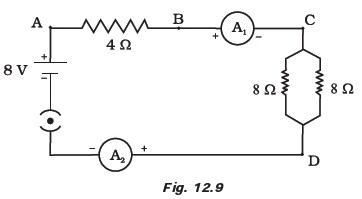## Answers to Multiple Choice Questions

Multiple Choice Questions